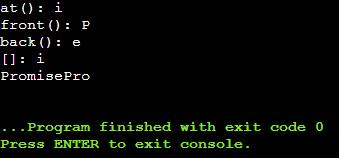# Substring Compare and Operators of String Class in C++

## Substring, Compare, and Operators of String Class in C++

In this article, I am going to discuss Substring, Compare, and Operators of String Class in C++ Language with examples. Please read our previous article, where we discussed Copy and Find Functions of String Class in C++ with examples.

##### String Class – Substring, Compare, and Operators-

Let us look at the function that is substring.

##### Substring (start, number):

This function will take out the part of a string. We can extract a portion of a string.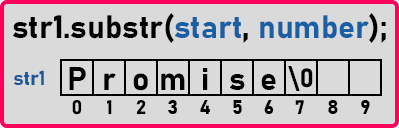Here we have an example that is the word ‘Promise’. Now we want to pick out a substring so we will write ‘str1.substr(3)’. It will take ‘mise’ that is it will take a string from 3rd index onwards of the main string. This function will return a string object. It will create a new String object and it will return that object. We can give the number of characters that we want in a substring. We will write it as ‘str1.substr(3, 5)’. It will take ‘mis’. Now let us look at the next function.

##### Compare (str):

We have already seen compare function that is ‘strcmp()’ in our previous article. In the same manner ‘str1.compare(str2)’ will work. It will also compare two strings in dictionary order and return the result in negative, zero, or positive.

1. If the first string is smaller than the second string then it will return a negative value.
2. If the first string is greater than the second string or it comes after than second then it will return a positive value.
3. If the first string is equal to the second string then it will return zero.

Now let us see these functions in a program.

##### Program for Substring, Compare functions of String Class in C++:
```#include <iostream>
#include <string.h>
using namespace std;
int main()
{
string str1 = "Promise";
string str2 = "Pro";
cout << "substr: " << str1.substr (3, 3) << endl;	//substr
cout << "substr: " << str1.compare (str2) << endl;;	//compare
}
```
###### Output: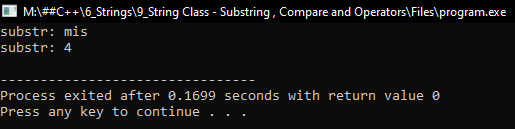##### C++ String class Operators:

I will show you some operators defined by the C++ String class.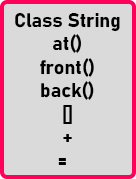##### at() function in C++: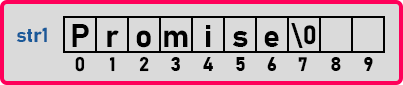This ‘at’ function will give a letter at a particular index. Here if say ‘str1.at(4)’ then it will return the ‘i’ character. So, we can find out a letter at a given index. It is just like using this subscript operator i.e. ‘[]’. If we write ‘str1’ then it will also return ‘i’. So, by using this operator we can read as well as we can write the letters present at a given index.

So, these two ‘at’ and ‘[]’ work in a similar way. But one interesting thing to observe here is that ‘at’ is a member function and string is a class, it is not an array. Then how ‘[]’ operator works on it. This is an overloaded operator. Inside C++ classes, we can overload operators also, we can define functions for operators or we can define a function as an operator. We will be learning operator overloading in upcoming articles.

##### front() and back() functions in C++These two are very simple functions. ‘front()’ will give the first letter of a string that is ‘P’ in this case and ‘back()’ will give you the last letter of a string that is ‘e’. Let us look at the plus operator.

##### ‘+’ Operator:

This ‘+’ operator will concatenate the two strings. For example,
string str1 = “Hello”;
string str2 = “World”;
string str3 = str1 + str2;
Here we are concatenating two strings with the help of the ‘+’ operator and assigning the result to another string object that is str3. Now if we print str3 then it will print “Hello World”. Now let us see all the above functions and operations in the C/C++ program.

##### String Class functions and operations Program in C++:
```#include <iostream>
#include <string.h>
using namespace std;
int main()
{
string str1 = "Promise";
string str2 = "Pro";

//at function
cout << "at(): " << str1.at (4) << endl;
//front function
cout << "front(): " << str1.front () << endl;
//back function
cout << "back(): " << str1.back () << endl;
//subscript opearator
cout << "[]: " << str1 << endl;